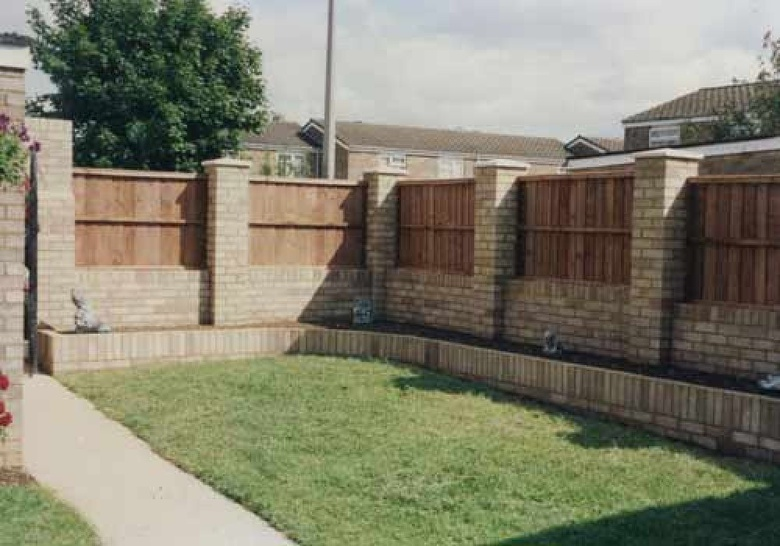Author:
Cindy
Subject:
Elementary Mathematics, Professional Learning
Material Type:
Activity/Lab
Level:
Upper Elementary
Tags:
Lesson Plan
Language:
English
Media Formats:
Text/HTML

# Education Standards## Overview

The purpose of this task is to explore multi-digit multiplication and lead to students discovering the distributive property.

# Summary

This is an Explore math task in the CMI framework to explore thinking about multiplication and the distributive property.

# Background for Teachers:

Priority Standard #1: Represent and Understand Multiplication and Division

Prior Grades: Develop an understanding of the meanings of multiplication and division and represent and solve multiplication and division problems within 100 (3.OA.1-6). By the end of Grade 3, know from memory all products of two one-digit numbers (3.OA.7.b).

4th Grade: Understand multiplication as comparison (4.OA.1-2). Use strategies based on place value and the properties of operations to multiply a whole number of up to four digits by a one-digit whole number, and multiply two, two-digit numbers (4.NBT.5). Use strategies based on place value, the properties of operations, and the relationship between multiplication and division to divide up to four-digit dividends and one-digit divisors (4.NBT.6).

# Student Prior Knowledge:

Learning Intentions:

• Students will be to explain their thinking in solving multi-digit multiplication problems.
• Students will explore the distributive property as a way to efficiently solve multiplication.

Success Criteria:

• Students will be able to share their thoughts and ideas on how to write multiplication equations for solving multi-digit multiplication problems (using the distributive property).

# Task: (Series of related problems)

Directions: First, create an array and area model to show your thinking. Then, see if you can find a way to solve without using a picture or model of any kind.

Problem #1:  You are building a wall 6 bricks high and 24 bricks long. How many bricks will you need to complete your wall?

Discussion:  Share models and strategies. Did anyone write an equation to solve with a model, array, or area model?

Give directions again: First, create an array and area model to show your thinking. Then, see if you can find a way to solve without using a picture or model of any kind.

Problem #2: Your neighbor is still really annoying, so you decided to make your wall 26 bricks high and 24 bricks long now. Now how may bricks will you need?

Discussion:  Share models and strategies. Did anyone write an equation to solve with a model, array, or area model?

Can students draw conclusions?  Is this a strategy that will always work?  Why or why not?

# Assessment during explore discussions and sharing. (This is done while students are working before class discussion).

Questions to ask to get students to think about what they are doing and to help them articulate their thinking.

• What strategy did you use?
• Can you show me where these are in your models?
• Where do these numbers come from?
• What relationships do you see?

# Exit ticket out the door: Post it note: Aha, Wondering, etc.

Since this is an explore task to have students discover thinking, there is not a paper assessment.  However it is appropriate to have students complete exit tickets.

1. Did they have an Aha?

2. Is there something they are wondering now?

3. Did they make a connection to something they hadn't before?

4. Are they still really confused?